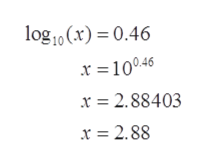Consider the equationlog x=0.46.Rewrite the equation using exponents instead of logs.    x=10∧  Estimate a solution for     x with a calculator.Round your answer to two decimal places.    x=

Question

Consider the equation

log x=0.46.

Rewrite the equation using exponents instead of logs.

x=10∧

Estimate a solution for     x with a calculator.

Round your answer to two decimal places.

x=

Step 1

To determine the exponential form of log x=0.46 and the value of x.

Step 2

Used formula:

Step 3

Apply the log formula on ...help_outlineImage Transcriptioncloselog10(x) 0.46 46 x 2.88403 x = 2.88 fullscreen

Want to see the full answer?

See Solution

Want to see this answer and more?

Our solutions are written by experts, many with advanced degrees, and available 24/7

See Solution
Tagged in

Algebra